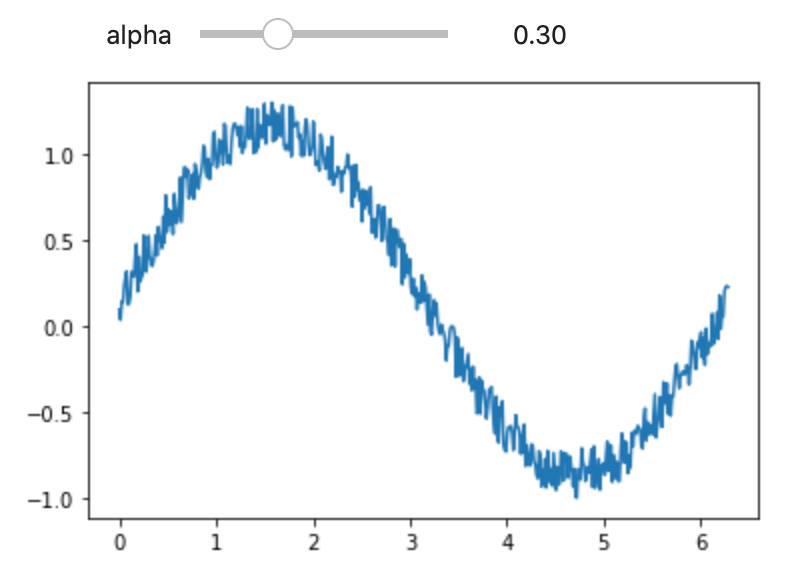October 22, 2021

# How to make interactive plots in Jupyter

Python has great options for plotting data. However, sometimes you want to explore data by changing parameters and rerunning plots to explore the effect of those changed parameters. This slows down the cycle of exploration. Luckily, Jupyter offers you a way to make you plots interactive, so you can see the effect of parameter changes immediately. Here is how to do it.

## Start jupyter

Before you proceed, start a jupyter notebook with a Python kernel where you can type in the code.

Next, you need a few imports:

``````%matplotlib inline
import numpy as np
from matplotlib import pyplot as plt
from ipywidgets import interactive, fixed``````

## Write a plotting function

Next, you have to provide a function with parameters you want to change. The function should plot you output. Here is a single example with a noise sine function, where we want the user to be able to change the noise level.

``````def noisy_sine(alpha=0.0):
n_samples = 500
alpha = np.abs(alpha)
x = np.linspace(0,2*np.pi,n_samples)
y = np.sin(x) + np.random.random(n_samples) * alpha
plt.plot(x, y)
plt.show()
return x,y``````

## Provide your function as input to interactive()

Finally, pass your function to interactive() along with value ranges for the parameters you want to explore.

``interactive(noisy_sine, alpha=(0.0, 1.0))``

The result should look like this:#### kostas

View all posts by kostas →

This site uses Akismet to reduce spam. Learn how your comment data is processed.